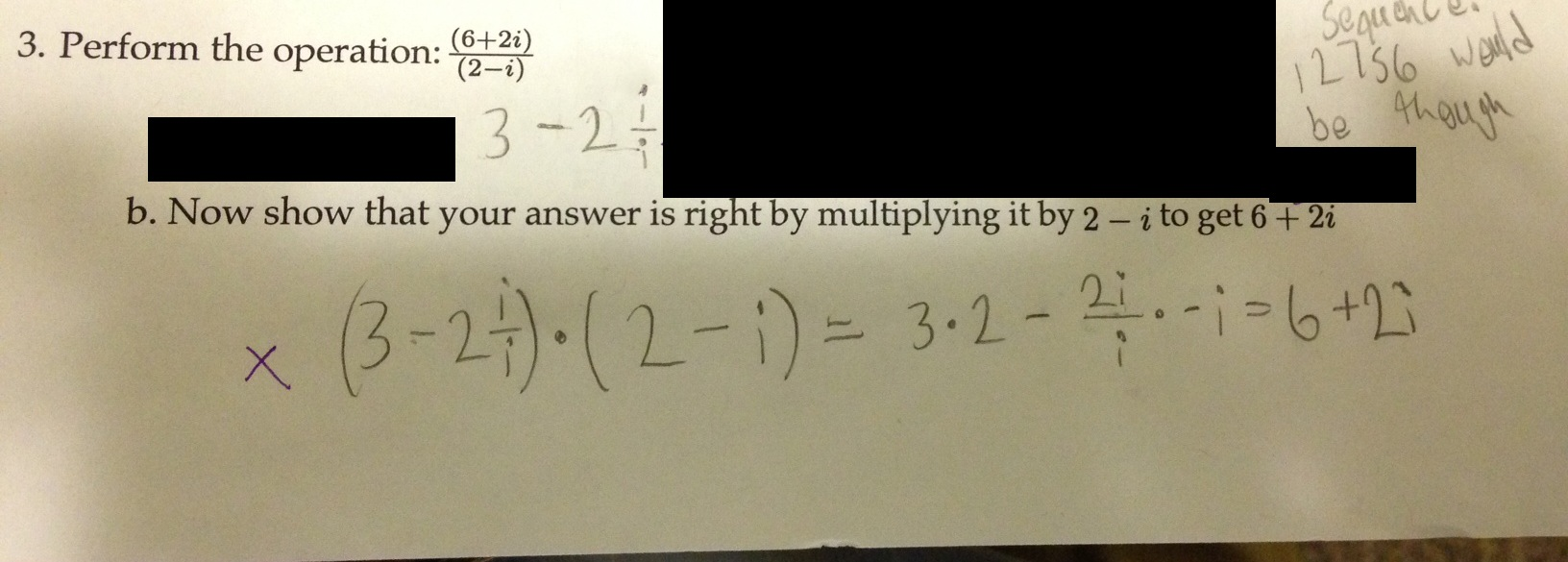Categories

# Complex DivisionThanks to John Burk for the submission. He writes, “I know this student wouldn’t simplify (6+2x)/(3-x) this way.”

So: if the student wouldn’t simplify (6+2x)/(3-x) this way, then how did the student end up messing it up for (6+2i)/(3-i)?

## 4 replies on “Complex Division”

How would you simplify$\dfrac {\Gamma \left( 6+2x\right) } {3-x}$ ? It’s not the same, because multiplying by complex conjugate doesn’t get rid of the x in the denominator. I think$\left( i\right) ^{2}$ is a different fish from$\left( x\right) ^{2}$
Also, the multiplication of the two binomials is a math mistake in its own right. Two for one, thanks!

sorry, no idea why that peculiar symbol is there, please ignore it. Just a LaTex learner.

Via Colleen, who commented on the picture on a different page: “This student assumes that (a+b)/(c+d) = a/b+b/d. We could show him that this doesn’t work with numbers?”

I agree with Colleen that we could use specific numbers to show (a+b)/(c+d) != a/c + b/d. However, that never sticks. How do we permanently knock this misunderstanding out of students minds?